# 滴~自动电梯邀请函已送达

2022-09-08  作者：我的世界官网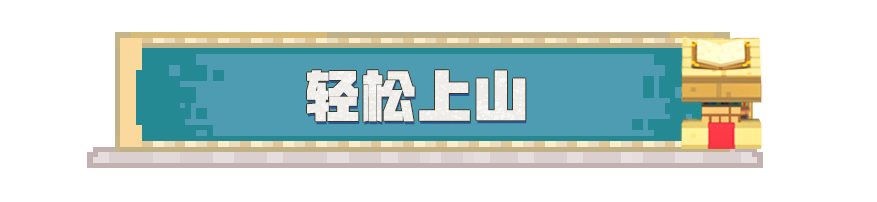/give @s command_block得到命令方块~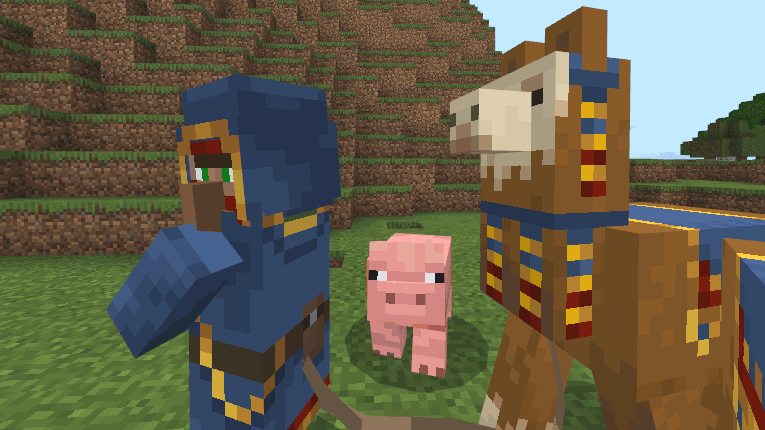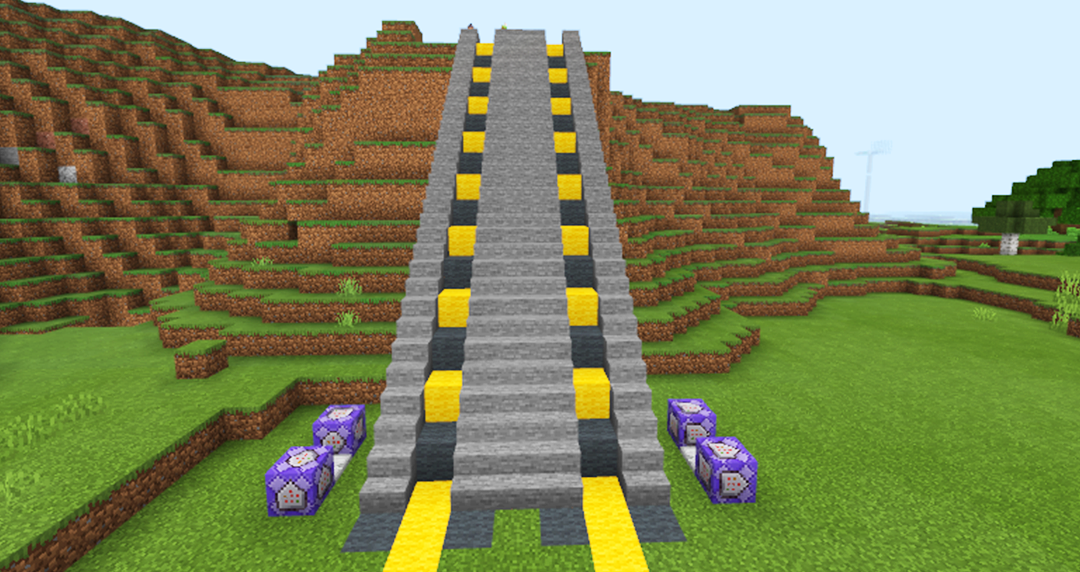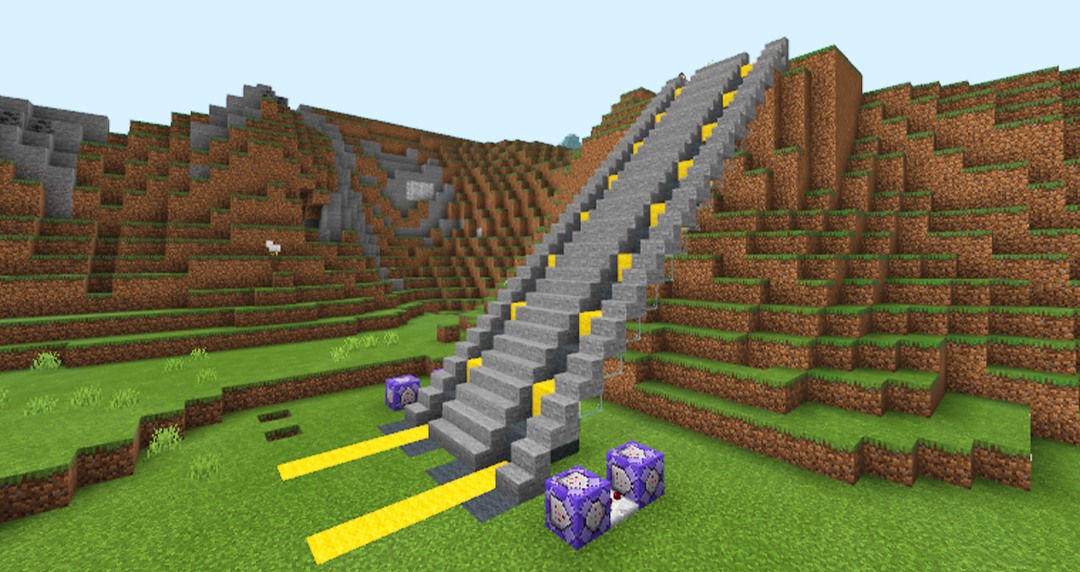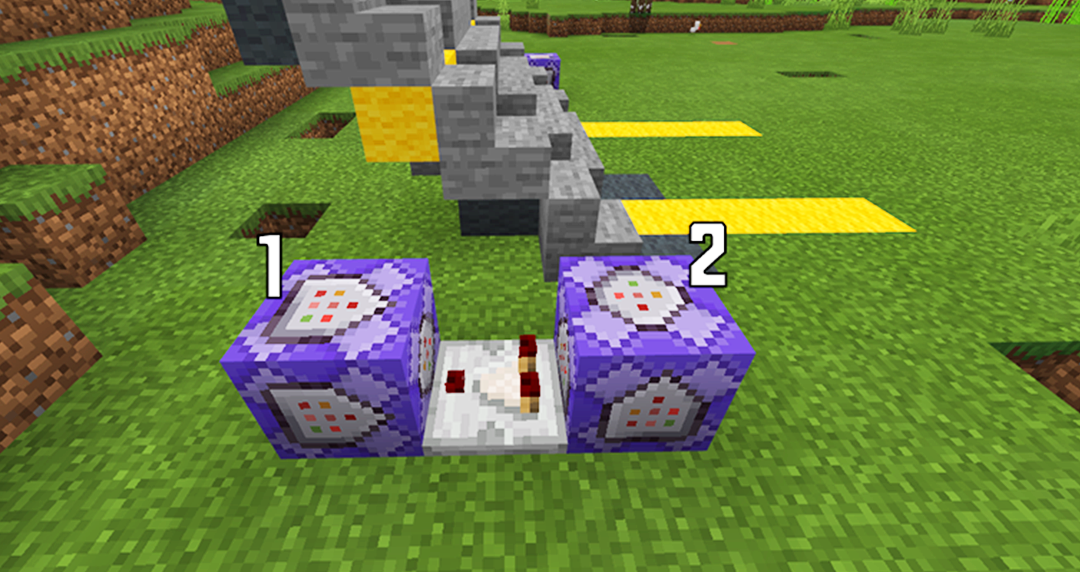/execute @r[x=X1,y=Y1,z=Z1, dx=X2-X1，dy=Y2-Y1，dz=Z2-Z1] ~~~ testforblock ~~-1~ wooldx=X2-X1，dy=Y2-Y1，dz=Z2-Z1，都是坐标数字相减得出的结果~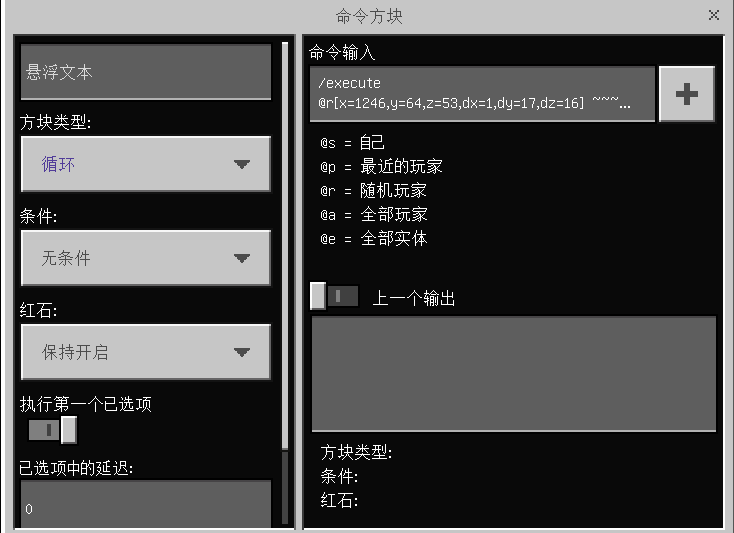/execute @r[x=X1,y=Y1,z=Z1, dx=X2-X1，dy=Y2-Y1，dz=Z2-Z1] ~~~ tp @p[x=X1,y=Y1,z=Z1, dx=X2-X1，dy=Y2-Y1，dz=Z2-Z1] ~~1~1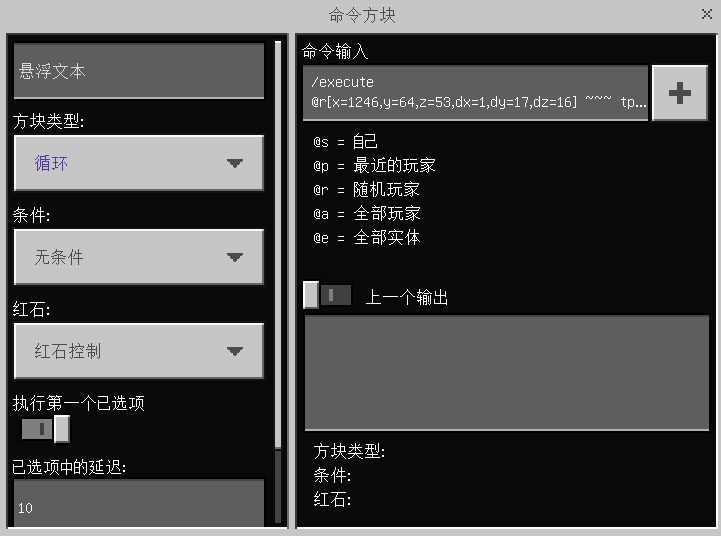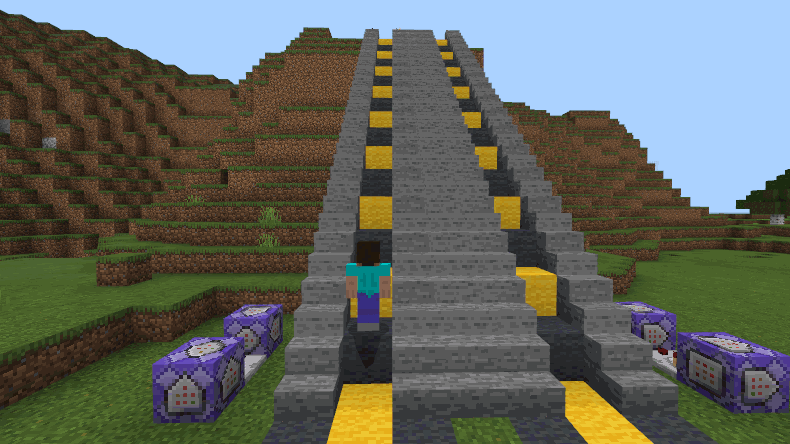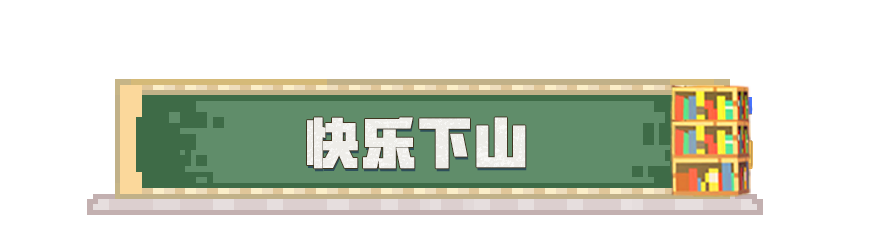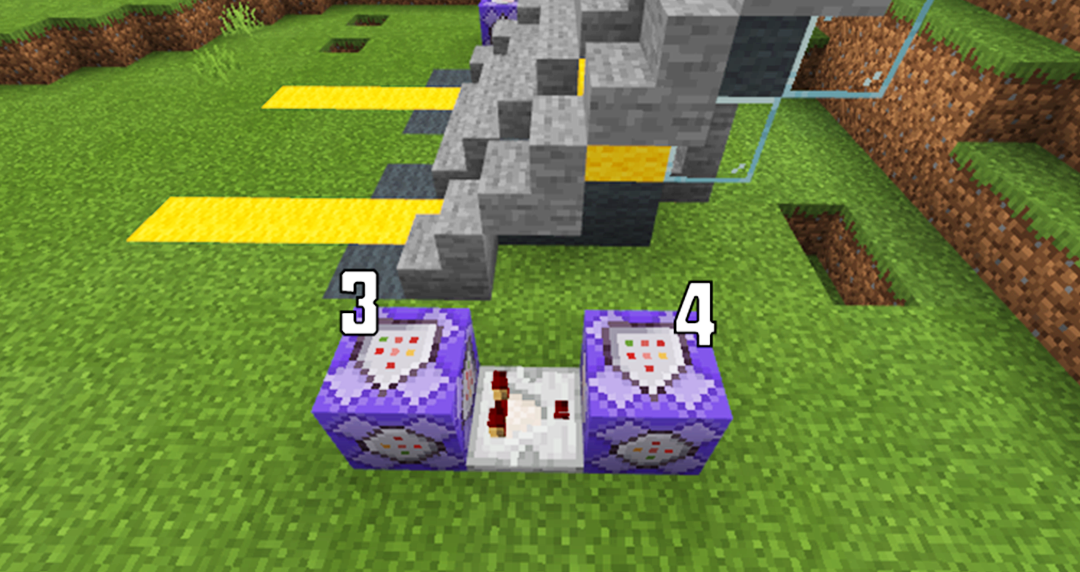/execute @r[x=X3,y=Y3,z=Z3, dx=X4-X3，dy=Y4-Y3，dz=Z4-Z3] ~~~ testforblock ~~-1~ wool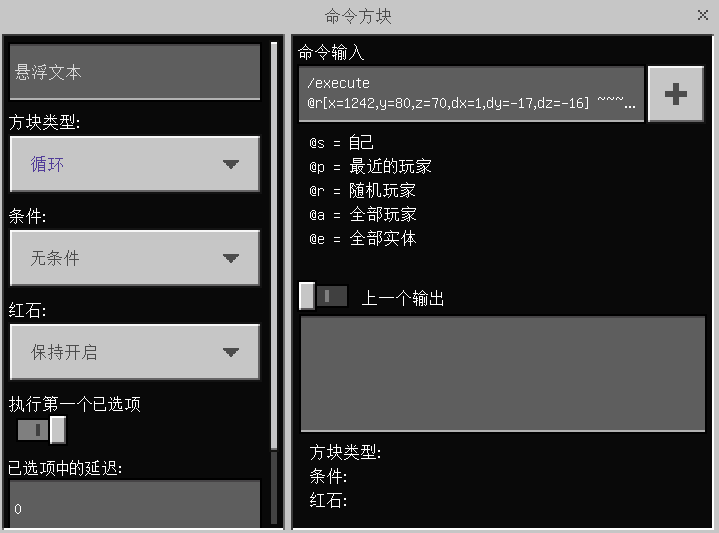/execute @r[x= X3,y=Y3,z=Z3, dx=X4-X3，dy=Y4-Y3，dz=Z4-Z3] ~~~ tp @p[x=X3,y=Y3,z=Z3, dx=X4-X3，dy=Y4-Y3，dz=Z4-Z3] ~~-1~-1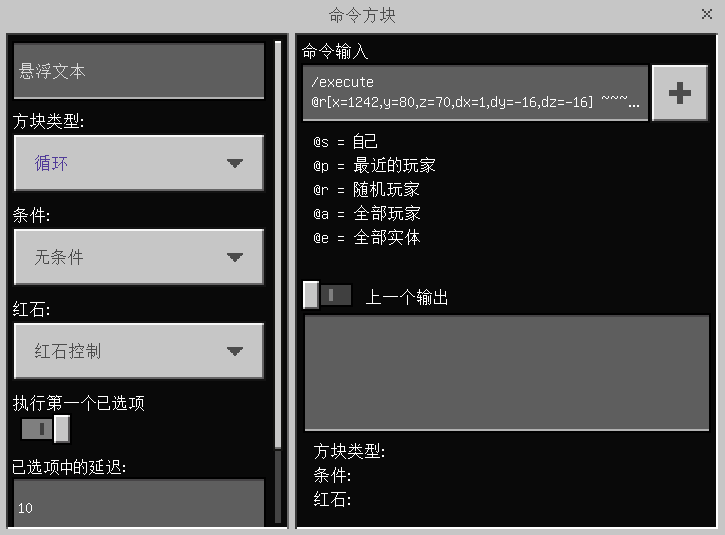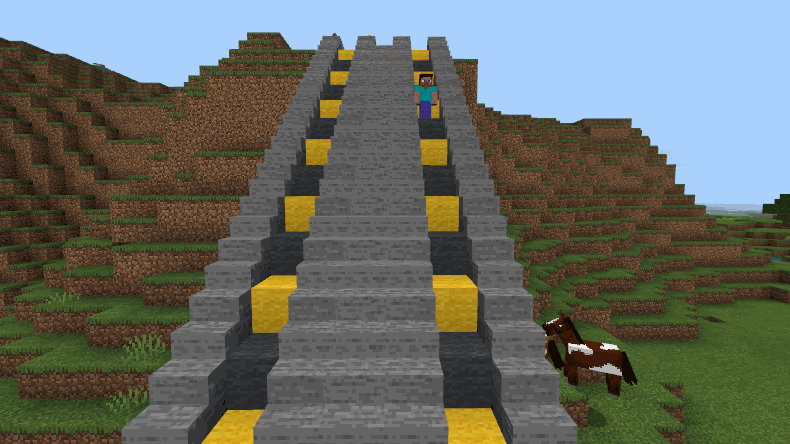×